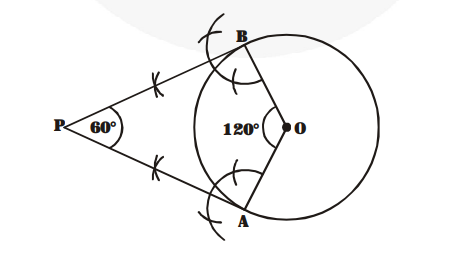# Draw a pair of tangents to a circle of radius 5 cm which are inclined to each other at an angle of 60°.

Question:

Draw a pair of tangents to a circle of radius 5 cm which are inclined to each other at an angle of 60°.

Solution:

Steps of construction :1. Draw circle with centre at O and radius 5 cm.

2. Construct radii $\mathrm{OA}$ and $\mathrm{OB}$ such that $\angle \mathrm{AOB}=120^{\circ}$.

3. Draw perpendiculars to OA and OB at A and B respectively and let they intersect at P.

Now, PA and PB is a pair of tangents inclined to each other at an angle of 60°.